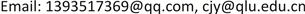1. 引言

2. 基于卷积神经网络和Softmax的蛋白质二级结构预测2.1. 卷积神经网络原理

s ( i , j ) = ( X * W ) ( i , j ) + b = ∑ k − 1 n _ i n ( X k * W k ) ( i , j ) + b (1)

2.2. Softmax回归模型

h θ ( x ) = 1 1 + exp ( − θ T x ) (2)

J ( θ ) = − 1 m [ ∑ i = 1 m y ( i ) log h θ ( x ( i ) ) + ( 1 − y ( i ) ) log ( 1 − h θ ( x ( i ) ) ) ] (3)

h θ ( x ( i ) ) = [ p ( y ( i ) = 1 | x ( i ) ; θ ) p ( y ( i ) = 2 | x ( i ) ; θ ) ⋮ p ( y ( i ) = k | x ( i ) ; θ ) ] = 1 ∑ j = 1 k e θ j T x ( i ) [ θ 1 T x ( i ) θ 2 T x ( i ) ⋮ θ k T x ( i ) ] (4)

θ = [ — θ 1 T — — θ 2 T — ⋮ — θ K T — ] (5)

Softmax回归所对应的损失函数如下所示：

J ( θ ) = − 1 m [ ∑ i = 1 m ∑ j = 1 k 1 { y ( i ) = j } log e θ j T x ( i ) ∑ l = 1 k e θ l T x ( i ) ] (6)

P ( y ( i ) = j | x ( i ) ; θ ) = e θ j T x ( i ) ∑ l = 1 k e θ j T x ( i ) (7)

2.3. 卷积神经网络和Softmax网络模型

3. 实验和分析

3.1. 蛋白质数据库

3.2. 实验过程

3.3. 实验结果及分析

Q3是被用于残基上的，通过计算正确预测的蛋白质残基占已知蛋白质二级结构序列中总的残基数的比例计算出。Q3值的范围为 [ 0 , 1 ] ，1表示准确预测。Q3就可以表示为：

Q 3 = 正 确 预 测 的 残 基 数 残 基 总 数 (8)

SOV方法是由Burkhard Rost  等人提出的一个基于重叠片段比值的测度。与Q3正确率的计算方法不同的是，SOV方法计算的是能够正确预测蛋白质二级结构的片段比例，从影响因素的角度来看，SOV会忽略一些蛋白质二级结构元素末端的小错误。

The results of convolutional neural networ
123平均

SOV73.2972.4272.0472.58

SOV72.6971.6171.6671.98

The results of convolutional neural network-Softma
123平均

SOV73.6274.4373.5673.87

SOV72.7973.1173.0872.99

4. 总结